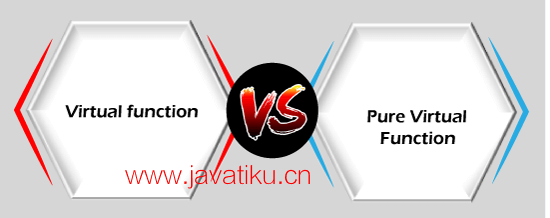# C++教程-C++虚函数vs纯虚函数### 什么是虚函数？

``````#include <iostream>
using namespace std;

class base
{
public:
void show()
{
std::cout << "Base class" << std::endl;
}
};

class derived1 : public base
{
public:
void show()
{
std::cout << "Derived class 1" << std::endl;
}
};

class derived2 : public base
{
public:
void show()
{
std::cout << "Derived class 2" << std::endl;
}
};

int main()
{
base *b;
derived1 d1;
derived2 d2;

b = &d1;
b->show();

b = &d2;
b->show();

return 0;
}``````

``````virtual void show()
{
std::cout << "Base class" << std::endl;
}``````

``````Derived class 1
Derived class 2``````

• 它是一种运行时多态性（runtime polymorphism）。
• 基类和派生类具有相同的函数名，将基类的指针赋值为派生类对象的地址时，指针将执行基类函数。
• 如果将函数声明为虚函数，那么编译器将根据指针分配给基类的地址在运行时确定要执行的函数。

### 什么是纯虚函数？

• 纯虚函数是一个“什么也不做”的函数。这里的“什么也不做”意味着它只提供了模板，派生类实现该函数。
• 纯虚函数可以看作是一个空函数，也就是说，相对于基类而言，纯虚函数没有任何定义。
• 程序员需要在派生类中重新定义纯虚函数，因为在基类中它没有定义。
• 含有纯虚函数的类不能用来直接创建对象。这意味着如果类包含任何纯虚函数，我们就不能创建该类的对象。这种类型的类被称为抽象类（abstract class）。

``virtual void display() = 0;``

``virtual void display() {}``

``````#include <iostream>
using namespace std;

// 抽象类
class Shape
{
public:
// 纯虚函数
virtual float calculateArea() = 0;
};

// 正方形类，继承自抽象类Shape
class Square : public Shape
{
float a;

public:
Square(float l)
{
a = l;
}

// 实现抽象类中的纯虚函数
float calculateArea()
{
return a * a;
}
};

// 圆形类，继承自抽象类Shape
class Circle : public Shape
{
float r;

public:
Circle(float x)
{
r = x;
}

// 实现抽象类中的纯虚函数
float calculateArea()
{
return 3.14 * r * r;
}
};

// 矩形类，继承自抽象类Shape
class Rectangle : public Shape
{
float l;
float b;

public:
Rectangle(float x, float y)
{
l = x;
b = y;
}

// 实现抽象类中的纯虚函数
float calculateArea()
{
return l * b;
}
};

int main()
{
Shape *shape;
Square s(3.4);
Rectangle r(5, 6);
Circle c(7.8);

// 将shape指针指向不同的派生类对象
shape = &s;
int a1 = shape->calculateArea();

shape = &r;
int a2 = shape->calculateArea();

shape = &c;
int a3 = shape->calculateArea();

// 输出计算出的面积
std::cout << "Area of the square is " << a1 << std::endl;
std::cout << "Area of the rectangle is " << a2 << std::endl;
std::cout << "Area of the circle is " << a3 << std::endl;

return 0;
}``````

### 虚函数和纯虚函数之间的差异如下：• 虚函数是基类中的成员函数，可以在派生类中重新定义。
• 包含虚函数的类不是抽象类（abstract class）。
• 在虚函数中，函数的定义在基类中提供。
• 包含虚函数的基类可以被实例化。
• 如果派生类不重新定义基类的虚函数，则对编译过程没有影响。
• 所有派生类可以选择性地重新定义虚函数。

• 纯虚函数是类中没有定义的虚函数。
• 包含纯虚函数的类是抽象类（abstract class）。
• 在纯虚函数中，函数的声明在基类中提供，而在派生类中实现。
• 包含纯虚函数的基类成为抽象类，无法实例化。
• 如果派生类不定义基类的纯虚函数，不会引发任何错误，但派生类也成为抽象类。
• 所有派生类必须定义纯虚函数。